# RD Sharma Solutions Chapter 9 Arithmetic Progression Exercise 9.2 Class 10 Maths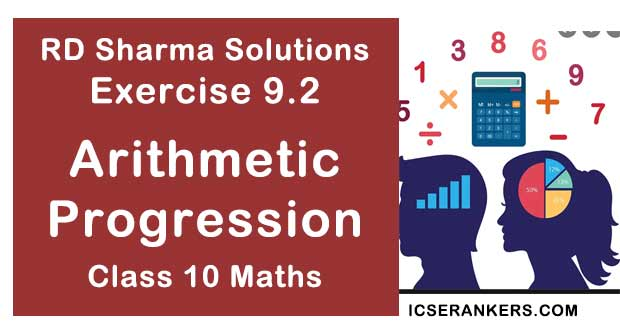Chapter Name RD Sharma Chapter 9 Arithmetic Progression Book Name RD Sharma Mathematics for Class 10 Other Exercises Exercise 9.1Exercise 9.3Exercise 9.4Exercise 9.5MCQ Related Study NCERT Solutions for Class 10 Maths

### Exercise 9.2 Solutions

1. For the following arithmetic progressions write the first term a and the common difference d:
(i) - 5, -1, 3,7, ........
(ii) 1/5, 3/5, 5/5, 7/5, .......
(iii) 0.3, 0.55, 0.80, 1.05, ......
(iv) -1.1, -3.1, -5.1, -7.1, .....

Solution

We know that if a is the first term and d is the common difference, the arithmetic progression is a, a +d, a + 2d + a + 3d, .......
(i) - 5, -1, 3,7, ........
Given arithmetic series is
- 5, -1, 3,7, ........
This is in the form of a, a + d, a + 2d + a + 3d, ...... by comparing these two
a = -5, a + d = 1, a + 2d = 3, a + 3d= 7, ........
First term (a) = -5
By subtracting second and first term, we get
(a + d) - (a) = d
-1-(-5) = d
4 = d
Common difference (d) = 4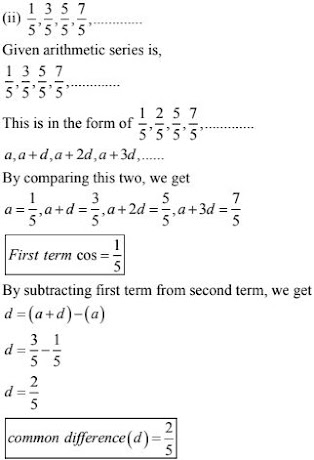(iii) 0.3, 0.55, 0.80, 1.05, .......
Given arithmetic series,
0.3, 0.55, 0.80,1.05, .....
General arithmetic series
a, a +d, a + 2d, a + 3d,....
By comparing,
a = 0.3, a + d = 0.5, a + 2d = 0.80, a + 3d = 1.05
First term(a) = 0.3
By subtracting first term from second term. We get
d = (a + d) - (a)
d = 0.55 - 0.3
d = 0.25
Common difference (d) = 0.25
(iv) -1.1, -3.1, -5.1, -7.1,......
General series is
a, a + d, a + 2d, a+ 3d, .....
By comparing this two, we get
a = - 1.1, a + d= -3.1, a + 2d= -5.1, a + 3d = -71
First term (a) = -1.1
Common difference (d) = (a + d) - (a)
= -3.1 - (-1.1)
Common difference (d) = -2

2. Write the arithmetic progressions write first term a and common difference d are as follows:
(i) a = 4, d = -3
(ii) a = -1, d = 1/2
(iii) a = -1.5, d = -0.5

Solution

We know that, if first term (a) = a and common difference = d, then the arithmetic series is, a, a+d, a+2d, a+3d, ......
(i) a = 4, d = -3
Given first term (a) = 4
Common difference(d) = -3
Then arithmetic progression is,
a, a+ d, a + 2d, a+ 3d, ......
⇒ 4, 4 - 3, a + 2(-3), 4 + 3(-3),.....
⇒ 4, 1, -2; -5, -8,.....

(ii) Given , First term(a) = -1
Common difference(d) = 1/2
Then arithmetic progression is,
⇒ a, a+d, a+ 2d, a+ 3d, .......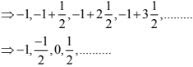(iii) Given, First term(a) = -1.5
Common difference(d) = -0.5
Then arithmetic progression is
⇒ a, a + d, a +2d, a + 3d,.....
⇒ -1.5, -1.5 - 0.5, -1.5+2(-0.5), -1.5 + 3(-0.5)
⇒ -1.5, -2, -2.5, -3,.....
Then required progression is
-1.5, -2, -2.5, -3,....

3. In which of the following situations, the sequence of numbers formed will form an A.P.?
(i) The cost of digging a well for the first metre is Rs 150 and rises by Rs 20 for each succeeding metre.
(ii) The amount of air present in the cylinder when a vacuum pump removes each time 1/4 of their remaining in the cylinder.

Solution

(i) Given,
Cost of digging a well for the first meter(c1 ) = Rs. 150.
Cost rises by Rs. 20 for each succeeding meter
Then,
Cost of digging for the second meter (c2 )= Rs. 150 + Rs 20 = Rs 170
Cost of digging for the third meter (c3 ) = Rs. 170 + Rs 20 = Rs 190
Thus, costs of digging a well for different lengths are 150, 170, 190, 210,......
Clearly, this series in in A.P.
With first term (a) = 150, common difference(d) = 20

(ii) Given,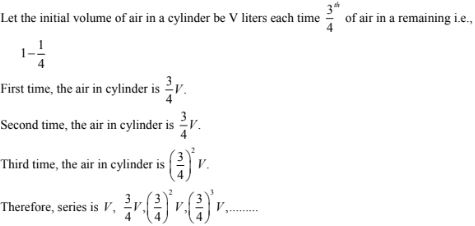4. Show that the sequence defined by an = 5n - 7 is an A.P., find its common difference.
Solution
Given sequence is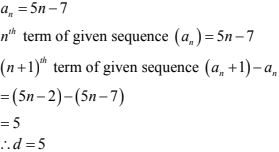5. Show that the sequence defined by an = 3n2 - 5 is not an A.P.
Solution
Given sequence is,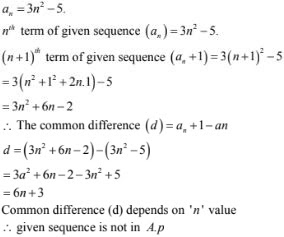6. The general term of a sequence is given by an = -4n + 15. Is the sequence an A.P. ? If so, find its 15th term and the common difference.
Solution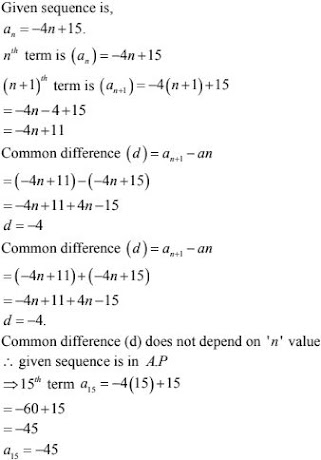7.  Find the common difference and write the next four terms of each of the following arithmetic progressions:
(i) 1, -2, -5, -8, .......
(ii) 0, -3, -6, -9, .......
(iii) -1, 1/4, 3/2,.......
(iv) -1, -5/6, -2/3,.......
Solution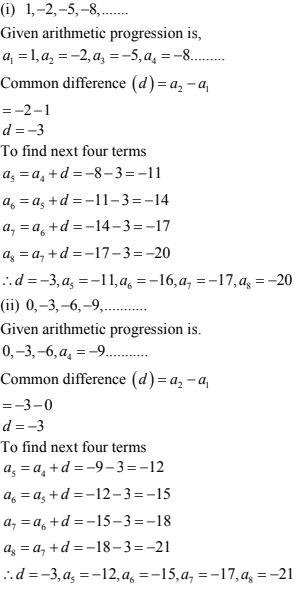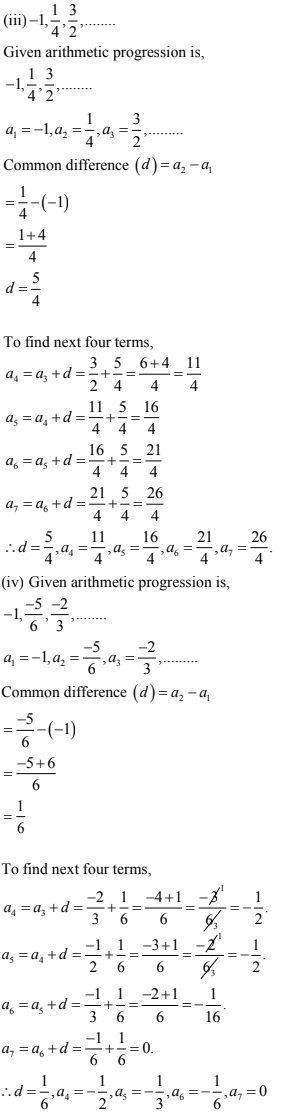8. Prove that no matter what the real numbers a and b are, the sequence with nth term a + nb is always an A.P. What is the common difference ?
Solution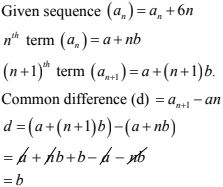∴ common difference (d) does not depend on nth value so, given sequence is in A.P. with (d) = b

9. Write the sequence with nth term:
(i) an = 3 + 4n
Solution
(i) an = 3 + 4n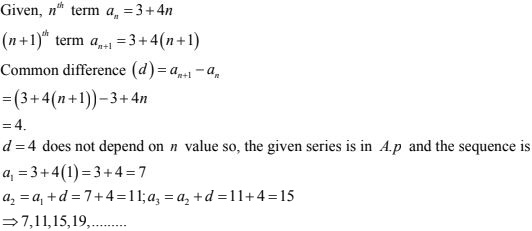(ii) an = 5 + 2n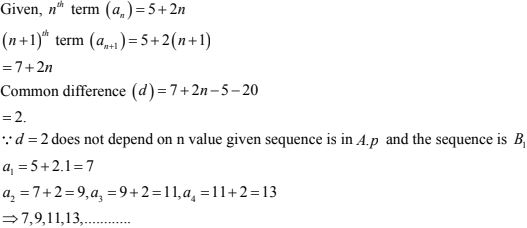(iii) an = 6 - n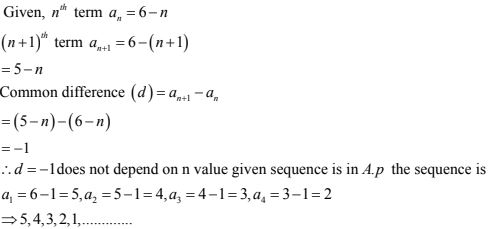(iv) an = 9 - 5n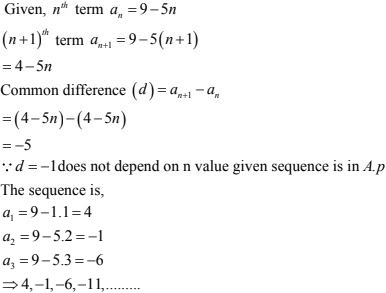10. Find out which of the following sequences are arithmetic progressions. For those which are arithmetic progressions, find out the common differences.
Solution
(i) 3, 6, 12, 24; .........
General arithmetic progression is a, a + d, a +2d, a + 3d, .......
Common difference (d) = Second term - first term
= (d + d) - a = d (or )
= Third term - second term
= (a + 2d) - (a +d) = d
To check given sequence is in A.P. or not we use this condition.
Second term - First term = Third term - Second term
a1 = 3, a2 = 6,  a3 = 12, a4 = 24
Second term - First term = 6 - 3= 3
Third term - Second term = 12 - 6 = 6
This two are not equal so given sequence is not in A.P.

(ii) 0, -4, -8, -12, .......
In the given sequence
a1 = 0, a2 = - 4, a3 = -8, a4 = -12
Check the condition
Second term - first term = third term - second term
a2 - a1 = a3 - a2
-4 - 0 = -8 - (-4)
-4 = + 8 + 4
-4 = -4
Condition is satisfied ∴ given sequence is in A.P. with common difference
(d) = a2 - a1 = - 4

(iii) 1/2, 1/4, 1/6, 1/8,.......
In the given sequence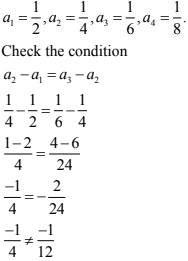Condition is not satisfied
∴ given sequence not in A.P.

(iv) 12, 2, -8, -18, .........
In the given sequence
a1 = 12, a2 = 2, a3 = -8, a4 = -18
Check the condition
a2 - a1 = a3 - a2
2 - 12 = -8 - 2
-10 = -10
∴ given sequence is in A.P. with common difference d = 10

(v) 3, 3, 3, 3, ........
In the given sequence
a1 = 3, a2 = 3, a3 = 3, a4 = 3
Check the condition
a2 - a1 = a3 - a2
3 - 3 = 3 - 3
0 = 0
∴ given sequence is in A.P. with common difference d = 0

(vi) P, P + 90, P + 80, P + 270, .......where P = (999)
In the given sequence
a1 = P, a2 = p + 90, a3 = p+180, a4 = P+ 270
Check the condition
a2 - a1 = a3 - a2
P + 90 - P = P + 180 - P - 90
90 = 180 - 90
90 = 90
∴ given sequence is in A.P. with common difference d = 90

(vii) 1.0, 1.7, 2.4, 3.1, ......
In the given sequence
a1 = 1.0, a2 = 1.7, a3 = 2.4, a4 = 3.1
Check the condition
a2 - a1 = a8 - a2
1.7 - 1.0 = 2.4 - 1.7
0.7 = 0.7
∴ The given sequence is in A.P with d = 0.7

(viii) -225, -425, -625, -825,.....
In the given sequence
a1 = -225, a2 = -425, a3 = -625, a4 = -825
Check the condition
a2 - a1 = a3 - a2
-425 + 225 = -625 +425
-200 = -200
∴ The given sequence is in A.P. with d = -200

(ix) 10, 10 + 25 , 10 + 26 , 10 + 27 , .........
In the given sequence
a1 = 10, a2 = 10 + 25 , a3 = 10 + 26 , a4 = 10 + 27
Check the condition
a2 - a1 = a3 - a2
10 + 25 - 10 = 10 + 26 - 10 + 25
25 ≠ 26 - 25
∴ The given sequence is not in A.P.